## Friday, November 14, 2008

### Irodov Problem 1.247Let us consider the forces acting on each body one by one.
Mass m1:
On mass m1 there are two forces acting in the vertical direction i) the force of gravity m1g pulling it downwards and ii) the normal reaction N from the surface stopping it from moving down into the surface. Since this mass has no acceleration in the vertical direction we have,In the horizontal direction, there are two forces acting on the mass i) the tension in the string T1 pulling it forward and ii) the force of friction between the surface and the mass, kN, resisting its motion on the surface. Let the acceleration of the mass be a. Then we have,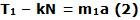Mass m2: There are two forces acting on this mass, i) the force of gravity m2g pulling it down and ii) the tension in the string T2 pulling it upwards. Its acceleration will be a the same as that of mass m1. So we have,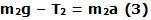The Cylindrical Pulley: Since the thread does not slip over the cylinder, each of the ends of the string induces a torque on it at its two ends namely T1R and T2R resulting in a net resultant torque of (T2-T1)R that is responsible for turning the cylinder and inducing angular accelerationin it. Since there is no slipping between the thread and the cylinder we have,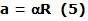Since the moment of inertia of the cylinder is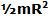we have,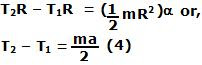Solving (1),(2),(3) and (4) we get,The work performed by friction force is simply -Nkx, where x is the distance traveled by mass m1 (the negative sign comes because the force of friction is in the opposite direction of that of the direction of motion of the mass m1). Now, since mass m1 moves with a constant acceleration a we have,